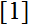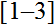# Volume 9, 2003, Number 3

Volume 9Number 1Number 2 ▷ Number 3 ▷ Number 4

Some characteristics of primes within modular rings
Original research paper. Pages 49—58
J. V. Leyendekkers and A. G. Shannon
Full paper (PDF, 3999 Kb) | Abstract

The squares of primes in the Modular Ring Z4 fall in even rows, R1, in Class ̅14 with R1 = 6Kj and Kj = ½ n(3n ± 1) (depending on the parity of j). The n values are found to equal the rows that the primes occupy when Z6 is set as a tabular array. The primes in Z4 equal a unique sum or difference of squares (x2 ± y2) and via n, the (x, y) pairs can be identified within the Z6 structure. The n values for composites follow well-defined linear functions that permit easy sorting. Finally, the parameter n in the well-known function P = h2n − 1 has been identified as the row in one or more of the Modular Rings Z10, Z6 or Z4 that contains one or more primes.

Properties of the restrictive factor
Original research paper. Pages 59—61
Laurențiu Panaitopol
Full paper (PDF, 1087 Kb) | Abstract

InKrassimir Atanassov has introduced the arithmetic function RF(n) defined by RF(1) = 1 and RF(n) = q1α1−1q2α2−1qkαk−1 whenever n = q1α1 q2α2qkαk, where q1, …, qk are pairwise different prime numbers and we have α1αk ≥ 1. The function RF(n) is called the restrictive factor. In the present paper we denote it simply by R(n).

A property of an arithmetic function
Original research paper. Pages 62—64
Krassimir T. Atanassov
Full paper (PDF, 903 Kb) | Abstract

A digital arithmetical function described inwill be defined and new its properties will be described.

Iterated Dirichlet series and the inverse of Ramanujan’s sum
Original research paper. Pages 65—72
Kenneth R. Johnson
Full paper (PDF, 2246 Kb) | Abstract

The theory of Dirichlet series having number theoretic functions of a single variable as coefficients has a rich history. In this paper we present a parallel theory for iterated Dirichlet series with number theoretic functions of two variables as coefficients and find the Dirichlet product inverse of Ramanujan’s sum. The results presented here are easily accessible to an Advanced Calculus or undergraduate Number Theory course.

Volume 9Number 1Number 2 ▷ Number 3 ▷ Number 4